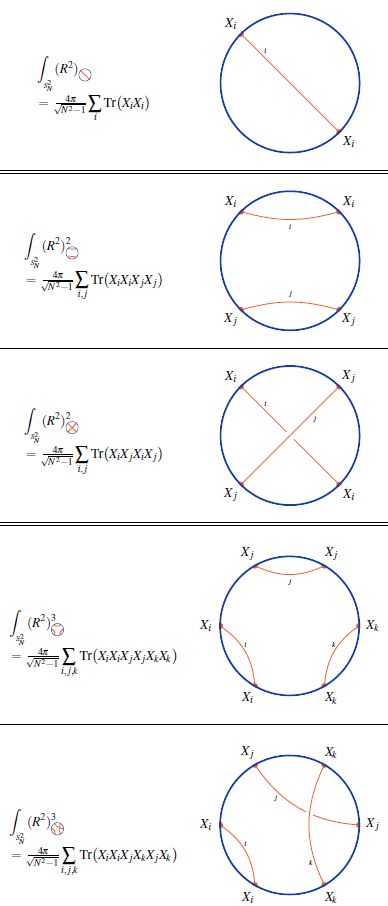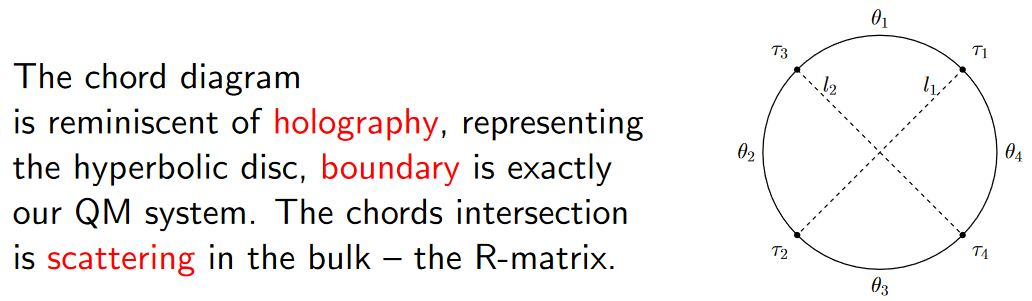Contents

# Contents

## Idea

In quantum field theory and statistical physics, a single trace operator is an observable/correlator on square matrix- or rather adjoint representation-valued scalar fields/random variables $\{\phi_i\}_{i \in I}$ (notationally subsuming derivatives of fields) which is built from the elementary field observables $\mathbf{\Phi}_i$ as a trace over the matrix product of a finite string of them:

(1)$\mathcal{O}_{i_1, \cdots i_n} \;=\; Tr\big( \mathbf{\Phi}_{i_1} \cdot \mathbf{\Phi}_{i_2} \cdot \cdots \cdot \mathbf{\Phi}_{i_n} \big)$

or more generally as linear combinations thereof.

If the fields $\phi_i$ indeed take values in the adjoint representation of a given gauge group, then the trace in the expression (1) ensures that this observable is gauge invariant. More general gauge invariant observables are hence multi-trace operators which are linear combinations of products of single-trace observables (i.e. polynomials of single-trace observables).

## Properties

### Correspondence to strings under AdS/CFT

Single trace operators/observables in conformal field theories such as super Yang-Mills theories play a special role in the AdS-CFT correspondence: They correspond to single string excitations on the AdS-supergravity side of the correspondence, where, curiously, the “string of characters/letters” in the argument of the trace gets literally mapped to a superstring in spacetime (see the references below).

From Polyakov 02, referring to gauge fields and their single trace operators as letter and words, respectively:

The picture which slowly arises from the above considerations is that of the space-time gradually disappearing in the regions of large curvature. The natural description in this case is provided by a gauge theory in which the basic objects are the texts formed from the gauge-invariant words. The theory provides us with the expectation values assigned to the various texts, words and sentences.

These expectation values can be calculated either from the gauge theory or from the strongly coupled 2d sigma model. The coupling in this model is proportional to the target space curvature. This target space can be interpreted as a usual continuous space-time only when the curvature is small. As we increase the coupling, this interpretation becomes more and more fuzzy and finally completely meaningless.

From Berenstein-Maldacena-Nastase 02, who write $Z$ for the elementary field observables (“letters”) $\mathbf{\Phi}$ above:

In summary, the “string of $Z$s” becomes the physical string and that each $Z$ carries one unit of $J$ which is one unit of $p_+$. Locality along the worldsheet of the string comes from the fact that planar diagrams allow only contractions of neighboring operators. So the Yang Mills theory gives a string bit model where each bit is a $Z$ operator.

On the CFT side these BMN operators of fixed length (of “letters”) are usefully identified as spin chains which, with the dilatation operator regarded as their Hamiltonian, are integrable systems (Minahan-Zarembo 02, Beisert-Staudacher 03).

This integrability allows a detailed matching between

under AdS/CFT duality (Beisert-Frolov-Staudacher-Tseytlin 03, …). For review see BBGK 04, Beisert et al. 10.

(…)

### Relation to weight systems on chord diagrams

For fields $\phi$ valued in a Lie algebra representations $C \in \mathfrak{g}Mod$ of a metric Lie algebra, with $\{\phi_a\}$ a linear basis for $C$ and $\{\phi^a\}$ denoting the dual basis, those single trace operators involving only metric pairs of fields, such as

$\mathcal{O} \;\coloneqq\; Tr_C \big( \mathbf{\Phi}_{a_1} \cdot \mathbf{\Phi}_{a_2} \cdot \mathbf{\Phi}^{a_1} \cdot \mathbf{\Phi}^{a_2} \big)$

(using Einstein summation convention) are equivalently values of Lie algebra weight systems on the chord diagram that expresses the index pairing.

Such binary pairings inside the trace are induced notably after statistical averaging in the SYK model and related systems, and has been argued to appear generically for dual observables of black hole thermodynamics (Berkooz-Narayan-Simón 18, Section 2.1).

For more on this see at weight systems on chord diagrams in physics.

## Examples

### Shape of D$p$$\bot$ D$(p+2)$-fuzzy funnelsgraphics from Sati-Schreiber 19c

We discuss how the single trace observables on the fuzzy 2-sphere-sections of Dp-D(p+2) brane intersection fuzzy funnels are given by su(2)-Lie algebra weight systems on chord diagrams (following Ramgoolam-Spence-Thomas 04, McNamara-Papageorgakis 05, see McNamara 06, Section 4 for review).

While in the commutative large N limit, all powers of the radius function on the fuzzy 2-sphere are equal

$\underset{N\to \infty}{\lim} \int_{S^2_N} R^{2 k} \;=\; 4 \pi \,;$

for finite $N$ there is an ordering ambiguity: In fact, the number of functions on the fuzzy 2-sphere at finite $N$ that all go to the same function $R^{2k}$ in the large N limit grows rapidly with $k$.

At $k = 1$ there is the single radius observable (?)

$\int_{S^2_N} R^2 \;=\; \int_{S^2_N} \underset{i}{\sum} X_i \cdot X_i \;=\; 4 \pi \tfrac{ N }{ \sqrt{N^2 -1} }$

At $k = 2$ there are, under the integral (?), two radius observables:

1. $\int_{S^2_N} \underset{i,j}{\sum} X_i X_i X_j X_j$

2. $\int_{S^2_N} \underset{i,j}{\sum} X_i X_j X_j X_i$

(Here we are using that under the integral/trace, a cyclic permutation of the factors in the integrand does not change the result).

Similarly for higher $k$, where the number of possible orderings increases rapidly. The combinatorics that appears here is familiar in knot theory:

Every ordering of operators, up to cyclic permutation, in the single trace observable $Tr(R^2)^n$ is encoded in a chord diagram and the value of the corresponding single trace observable is the value of the su(2)-Lie algebra weight system on this chord diagram.

(…)

### Correspondence to string excitations under AdS/CFT

The correspondence of single trace operators to superstring excitations under the AdS-CFT correspondence originates with these articles:

The identification of the relevant single trace operators with integrable spin chains is due to

which led to more detailed matching of single trace operators to rotating string excitations in

Review includes

### Relation to weight systems on chord diagrams

A general relation between single trace operators for observables on black hole thermodynamics and Lie algebra weight systems on chord diagrams (though not using the term “weight system”) is proposed in

For more on this see at weight systems on chord diagrams in physics.

#### For JT-gravity/SYK model

Discussion of (Lie algebra-)weight systems on chord diagrams encoding SYK model single trace observables:

• Antonio M. García-García, Yiyang Jia, Jacobus J. M. Verbaarschot, Exact moments of the Sachdev-Ye-Kitaev model up to order $1/N^2$, JHEP 04 (2018) 146 (arXiv:1801.02696)

• Micha Berkooz, Prithvi Narayan, Joan Simón, Chord diagrams, exact correlators in spin glasses and black hole bulk reconstruction, JHEP 08 (2018) 192 (arxiv:1806.04380)

following:

• László Erdős, Dominik Schröder, Phase Transition in the Density of States of Quantum Spin Glasses, D. Math Phys Anal Geom (2014) 17: 9164 (arXiv:1407.1552)

which in turn follows

• Philippe Flajolet, Marc Noy, Analytic Combinatorics of Chord Diagrams, pages 191–201 in Daniel Krob, Alexander A. Mikhalev,and Alexander V. Mikhalev, (eds.), Formal Power Series and Algebraic Combinatorics, Springer 2000 (doi:10.1007/978-3-662-04166-6_17)With emphasis on holographic content:

• Micha Berkooz, Mikhail Isachenkov, Vladimir Narovlansky, Genis Torrents, Section 5 of: Towards a full solution of the large $N$ double-scaled SYK model, JHEP 03 (2019) 079 (arxiv:1811.02584)

• Vladimir Narovlansky, Slide 23 (of 28) of: Towards a Solution of Large $N$ Double-Scaled SYK, 2019 (pdf)

and specifically in relation to Jackiw-Teitelboim gravity: